Home > INT3 > Chapter 7 > Lesson 7.2.4 > Problem7-95

7-95.
1. Without using a calculator, solve each equation. Homework Help ✎

1. log2(x) = 10

2. log3(x + 1) = 5

3. log3(3x) = 4

4. 4log4(x) = 7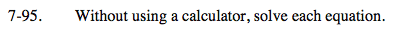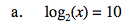Rewrite this equation in exponential form.

$x=2^{10}$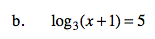x = 242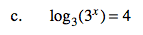Rewrite this equation in exponential form.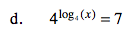x = 7